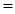# Water can be decomposed to its elements, H 2 and O 2 , using electrical energy or in a series of chemical reactions. The following sequence of reactions is one possibility: CaBr 2 (s) + H 2 O(g) → CaO(s) + 2 HBr(g) Hg( ℓ ) + 2 HBr(g) → HgBr 2 (s) + H 2 (g) HgBr 2 (s) + CaO(s) → HgO(s) + CaBr 2 (s) HgO(s) → Hg( ℓ ) + ½ O 2 (g) (a) Show that the net result of this series of reactions is the decomposition of water to its elements. (b) If you use 1000. kg of water, what mass of H 2 can be produced? (c) Calculate the value of Δ r H ° for each step in the series. Are the reactions predicted to be exo- or endothermic? Δ f H ° [CaBr 2 (s)] = –683.2 kJ/mol Δ f H ° [HgBr 2 (s)] = –169.5 kJ/mol (d) Comment on the commercial feasibility of using this series of reactions to produce H 2 (g) from water.### Chemistry & Chemical Reactivity

9th Edition
John C. Kotz + 3 others
Publisher: Cengage Learning
ISBN: 9781133949640

#### Solutions

Chapter
Section### Chemistry & Chemical Reactivity

9th Edition
John C. Kotz + 3 others
Publisher: Cengage Learning
ISBN: 9781133949640
Chapter 5, Problem 108SCQ
Textbook Problem
1 views

## Water can be decomposed to its elements, H2 and O2, using electrical energy or in a series of chemical reactions. The following sequence of reactions is one possibility:CaBr2(s) + H2O(g) → CaO(s) + 2 HBr(g)Hg(ℓ) + 2 HBr(g) → HgBr2(s) + H2(g)HgBr2(s) + CaO(s) → HgO(s) + CaBr2(s)HgO(s) → Hg(ℓ) + ½ O2(g)(a) Show that the net result of this series of reactions is the decomposition of water to its elements.(b) If you use 1000. kg of water, what mass of H2 can be produced?(c) Calculate the value of ΔrH° for each step in the series. Are the reactions predicted to be exo- or endothermic?ΔfH° [CaBr2(s)] = –683.2 kJ/molΔfH° [HgBr2(s)] = –169.5 kJ/mol(d) Comment on the commercial feasibility of using this series of reactions to produce H2(g) from water.

(a)

Interpretation Introduction

Interpretation:

To show the net result is the decomposition of water

Concept Introduction:

Heat energy required to raise the temperature of 1g of substance by 1K.Energy gained or lost can be calculated using the below equation.

q=C×m×ΔT

Where, q= energy gained or lost for a given mass of substance (m), C =specific heat capacity, ΔT= change in temperature.

The standard molar enthalpy of formation is the enthalpy change ΔfH0 is the enthalpy change for the formation of 1mol of a compound directly from its component elements in their standard states. And is given by

ΔrH0ΣnΔfH0(products)-ΣnΔfH0(reactants)

### Explanation of Solution

To show the net result is the decomposition of water.

Equation 1-2=A

A=CaBr2+H2O+HgCaO+HgBr2+H2

Equation A-3=

(b)

Interpretation Introduction

Interpretation:

If 1000Kg of water is used then identify the mass of H2 produced

Concept Introduction:

Heat energy required to raise the temperature of 1g of substance by 1K.Energy gained or lost can be calculated using the below equation.

q=C×m×ΔT

Where, q= energy gained or lost for a given mass of substance (m), C =specific heat capacity,ΔT= change in temperature.

The standard molar enthalpy of formation is the enthalpy change ΔfH0 is the enthalpy change for the formation of 1mol of a compound directly from its component elements in their standard states. And is given by

ΔrH0ΣnΔfH0(products)-ΣnΔfH0(reactants)

(c)

Interpretation Introduction

Interpretation:

The enthalpy of reaction has to be calculated.

Concept Introduction:

Heat energy required to raise the temperature of 1g of substance by 1K.Energy gained or lost can be calculated using the below equation.

q=C×m×ΔT

Where, q= energy gained or lost for a given mass of substance (m), C =specific heat capacity,ΔT= change in temperature.

The standard molar enthalpy of formation is the enthalpy change ΔfH0 is the enthalpy change for the formation of 1mol of a compound directly from its component elements in their standard states. And is given by

ΔrH0ΣnΔfH0(products)-ΣnΔfH0(reactants)

(d)

Interpretation Introduction

Interpretation:

Comment on the feasibility of using such a series of reaction to produce H2

Concept Introduction:

Heat energy required to raise the temperature of 1g of substance by 1K.Energy gained or lost can be calculated using the below equation.

q=C×m×ΔT

Where, q= energy gained or lost for a given mass of substance (m), C =specific heat capacity,ΔT= change in temperature.

The standard molar enthalpy of formation is the enthalpy change ΔfH0 is the enthalpy change for the formation of 1mol a compound directly from its component elements in their standard states. And is given by

ΔrH0ΣnΔfH0(products)-ΣnΔfH0(reactants)

### Still sussing out bartleby?

Check out a sample textbook solution.

See a sample solution

#### The Solution to Your Study Problems

Bartleby provides explanations to thousands of textbook problems written by our experts, many with advanced degrees!

Get Started

Find more solutions based on key concepts
In adults with vitamin D deficiency, poor bone mineralization can lead to pellagra. pernicious anemia. scurvy. ...

Nutrition: Concepts and Controversies - Standalone book (MindTap Course List)

Does the cell cycle refer to mitosis as well as meiosis?

Human Heredity: Principles and Issues (MindTap Course List)

4. The normal arterial range is

Cardiopulmonary Anatomy & Physiology

Consider the circuit in Figure 27.17 and assume the battery has no internal resistance. (i) Just after the swit...

Physics for Scientists and Engineers, Technology Update (No access codes included)

How may gyres exist in the world ocean?

Oceanography: An Invitation To Marine Science, Loose-leaf Versin